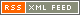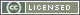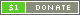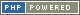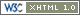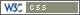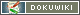# Summary Precedence Table

The table below shows all the Operators that PTPScript supports, according to their precedence (importance). The Operators with highest precedence (most important) are at the top.

Operators Description
Constants, Numbers, Strings, and Variables Individual terms
. .. Glued terms
[ ] ( ) Grouped terms
++ -- Auto-increment
** Exponentiation
+ - ? ! ~ @ +^ ~^ ?^ ??? Symbolic unary
* / % ~* @* +& ~& ?& +< ~< +> ~> Multiplication and division
+ - ~ @ +| ~| ?| +^ ~^ ?^ Addition and subtraction
destroy typeof abs cos log ln sin tan Named unary
... Range
< <= > >= lt le gt ge in Relational comparison
== != <> === !== eq ne is Equality comparison
&& || ^^ |?| Tight logical comparison
=
**= *= /= %= ~*= @*= +&= ~&= ?&= +<= ~<= +>= ~>=
+= -= ~= @= +|= ~|= ?|= +^= ~^= ?^=
...=
&&= ||= ^^= |?|=
Assignment
not and or xor err Loose logical
, : Group separators